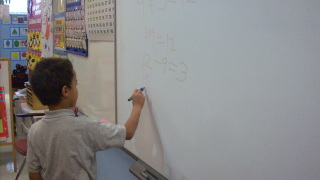# Identify Related Facts - Day 1 of 2

37 teachers like this lesson
Print Lesson

## Objective

SWBAT identify related addition and subtraction facts within 20.

#### Big Idea

It's all in the family! In this lesson, students will begin to identify and understand the relationship between addition and subtraction sentences.

## Activating Strategy

10 minutes

I begin by watching the following video, a song about fact families:

I then review the term related fact.  We have a discussion about the word related, and talk about how the people in their family are related.  The idea is to build the connection that related means that something is connected in some way.

I then have the students use connecting cubes to make a cube train to model an addition sentence.  Again, having them use 2 different colors.  I then have the students turn the cube train model to show the related addition fact.  For example:  2 + 3 = 5.

I like to have the students use connecting cubes so that they can see the concrete model of the relationship between the addition and subtraction sentences.  This is an important aspect of first grade, moving from the concrete to the abstract perspective of mathematical practices.

In order to get them to understand that these facts are related, I ask them if they solve one addition fact, how do they know the sum for the same numbers in a different order.  This will get them thinking about the commutative property, regardless of the order of the addends, the sum is always the same.

## Teaching Strategies

20 minutes

I write the following word problem on the board/chart paper:  (also available as a PPT Identify Related facts)

Jan has 4 pencils.  Matt gives her 9 more pencils.  How many pencils does Jan have?

I instruct the students to use connecting cubes to model the problem.  I guide the discussion using the following questions throughout the activity:

• What do we need to find? (How many pencils Jan has)
• How can we use cubes to show our addition sentence?  (I can put 4 red cubes and 9 blue cubes)  I would then write the sentence on the board/chart paper.
• What is another related addition sentence that we can write? I would then write that one on the board as well.
• How can we use our cube train to show a related subtraction problem? (I can take off the 9 blue cubes from the cube train.)  Write this subtraction sentence on the board.
• What is another related subtraction sentence?  Write that one as well.

I like to continue through these steps to model a few more fact families.

Guided Practice: (located on the second slide of Identify Related facts)

I like to have some addition and subtraction problems ready and have students identify the related facts.

Prior to moving onto independent practice, I have shoulder partners share why one of the example facts is not a fact family.

## Independent Practice

30 minutes

I use the Identify related facts worksheet for independent practice.

In this video, you will see a student using counters to help him to model the math problems:

For struggling students, I make a set of 5 addition and 5 subtraction related fact cards (using index cards) and we play memory to help them identify the related facts.

## Closing/Summarizing

5 minutes

To close out the lesson, I write three numbers on the board and call on random students to come up and demonstrate how to write an addition or subtraction sentence using the numbers.

In this picture, you will see the student writing related addition and subtraction sentences: